• +91 9723535972
• info@interviewmaterial.com

# Dice Reasoning Interview Questions and Answers

Question - 1 : -
Introduction- Dice Reasoning Questions
Types of  Dice

There are two types of dies i.e Standard Dice and Non-Standard Dice. This topic of Dice Reasoning Questions And Answers included both types of questions that were asked in the competitive exam i.e standard and non-standard

1. Standard Dice
Dice in which the sum of digits of opposite faces is always “7”
1+6 = 7
2+5 = 7
3+4 =7

2. Non-standard Dice
Non-standard dice are totally different from standard dies. Dice which does not follow the position of numbers of standard dice is called non-standard dice

Question - 2 : -
Tips and Tricks to Solve Dice Reasoning Questions

If you are solving Dice Reasoning Questions And Answers, you should know basic tips and tricks to solve Dice Reasoning Questions that help to understand closed and open dice reasoning questions clearly.
Trick Number 1:
Two opposite faces of dice cannot be adjacent to each other
Ex:  below figure, there are different positions of two dice are shown.  which number will appear on the face opposite to the number 4?

Solution:
Faces with four numbers i.e. 5, 3, 6, and 2 Are adjacent to the face with no. 4.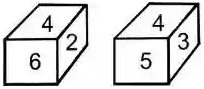Hence the number of faces 5, 3, 6, and 2 are cannot be opposite to the number 4.
Trick Number 2:
If two different positions of a dice are shown in the figure, and there are two common faces that appear is in the same position then the other remaining faces will be opposite to each other.
Ex: Below figure shows Two different positions of a dice.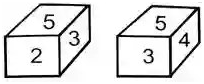Solution:
Here in both figures, the positions of two faces 5 and 3 are common.
And the remaining faces are 2 and 4.
Therefore, the number on the faces of numbers 2 and 4 are opposite to each other.
Trick Number 3:
If two different positions of a dice are shown, and only one face has the same position and same number, then each of the remaining faces is opposite to the remaining faces of the other dice.
Ex: Here figure shows there are both positions and number is common i.e. 3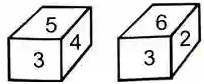Solution:
Hence,  the opposite of 2 is 4 and the opposite of 6 is 5.
Trick Number 4:
If two different positions of a dice are shown, and there is only one common number but the face position is different in this case, the opposite face of the common face is not showing in any two positions of dice. It means both dice show five faces and one face which hides. the face which is hidden is the opposite of the common face number.
Ex: Figure Shows two different positions of dice with common number 1, but it is not in the same position.
The hidden face with the number 6 is not shown.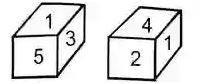Solution:
Hence, The hidden face is 6 who is opposite to the number 1.
Therefore, the opposite of face 3 is 2, and the opposite of face 5 is 1
Trick Number 5:
If two dies have only one symbol or sign is common but on different faces then the remaining faces will be opposed by the use of open dice as shown in the figure below.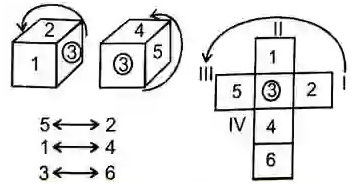5 opposite 2
1 opposite 4
3 opposite 6

Question - 3 : -
Find the Sign who will be on the face opposite to the face of the symbol *?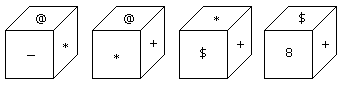(A) @
(B)  \$
(C) 8
(D) +

(C)
Solution:
Lets solve last two figure, in this \$ and + sign are common,
Hence remaining is * and 8. i.e opposite to each other

Question - 4 : -
Two positions of dies of the figure are shown below. find how many points will appear on the face opposite of face 5 points?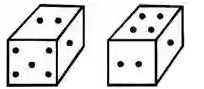(A) 3
(B) 2
(C) 1
(D) 4

Solution: In the above two positions of dies, there are two common faces i.e points 1 and 2.
so the remaining faces are opposite to each other
Therefore according to Trick number (2), There will be 4 points to the opposite of 5 point face.

Question - 5 : -
Which digit will appear on the face opposite to the face of number 4?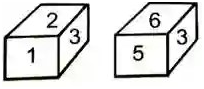(A) 3
(B) 6
(C) 5
(D) 2/3

Solution: Here are the common faces number is 3, and it is in the same position.  Hence  6  is opposite to 2  and 1 is opposite to 5.  Therefore opposite of 4 is 3.

Question - 6 : -
Two different positions of a dice are shown below. find out which number will appear on the face opposite to the face of number 5?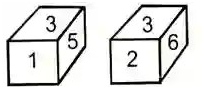(A) 2
(B) 2/6
(C) 6
(D) 5

Solution: According to Trick number (3). 3 is a common face,  and it is in the same position.
Therefore the number of the opposite face with the number 5 is 6.

Question - 7 : -
How many points will appear on the face opposite to 2  points?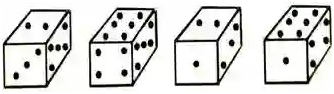(A) 6
(B) 3
(C) 2
(D) 4

Solution: In the first two positions of dice one face is common with their position i.e. 5.
Therefore, according to trick number (3), The face opposite to the face which contains 2 points is 6point.

Question - 8 : -
Which number appears on the face opposite of 6?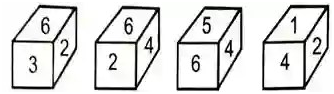(A) 3
(B) 2
(C) 1
(D) 4

Solution: In Figures 1st and 4th, there are two common numbers i.e. 4 and 2.
so the remaining side 6 and 1 are opposite to each other.

Question - 9 : -
Here below 4  positions of a cube are shown. Find out the sign who will be opposite to face ‘+’?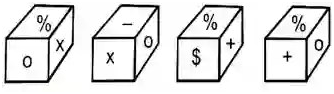(A) X
(B) %
(C) –
(D) \$

Solution: From given positions of  1 and 3, the common faces with is % and is in the same position. Hence according to trick number (3), the sign opposite to + is X.

Question - 10 : -
Four positions of a dice are shown below figure.  which number of the face will be opposite to the face of number 3?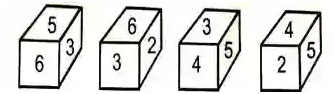(A) 5
(B) 2
(C) 1
(D) 4

Solution: The number of the adjacent faces to the face with number 3 is 5, 6, 2, and 4. Hence the opposite face with number 3 Will be opposite to the face with number 1

Name:
Email:

# Latest News# 10000+ interview questions in different categories